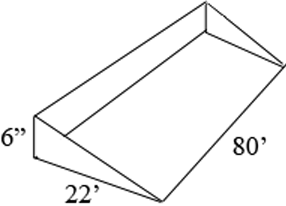SEARCH HOMEMath Central Quandaries & QueriesQuestion from Harold, a parent: I need to slope a area of my yard away from my house and garage. The length of my house is 80 feet long and i need to slope it 22 feet away. I want to raise the dirt at the foundation by 6 inches and slope it down to nothing at the width of 22 feet. How much soil would i require?Hi Harold,

I drew a rough sketch of the three dimensional region you want to fill with soil.The volume of this region is the area of the triangular cross-section, times the length of 80 feet. To obtain the volume in cubic feet you need all the dimensions in feet and hence the volume is

$\frac{22}{2} \times \frac{1}{2} \times 80 = 440 \mbox{ cubic feet.}$

There are 27 cubic feet in a cubic yard so if you want the volume in cubic yards divide 440 by 27.

I hope this helps,
PennyMath Central is supported by the University of Regina and the Imperial Oil Foundation.